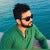# Maximum Swap

You are given an integer `num`. You can swap two digits at most once to get the maximum valued number.Return the maximum valued number you can get.

Example 1:

Example 2:

# Find the minimum number of swaps to sort unsorted array.

You are given an unordered array consisting of consecutive integers [1, 2, 3, …, n] without any duplicates. You are allowed to swap any two elements. Find the minimum number of swaps required to sort the array in ascending order.

Example

Perform the following steps:

# Reverse Vowel of a String.

Given a string `s`, reverse only all the vowels in the string and return it.

The vowels are `'a'`, `'e'`, `'i'`, `'o'`, and `'u'`, and they can appear in both cases.

Example 1:

Example 2:

Constraints:

• `1 <=…`

# Maximum Number of vowels in a substring of given Length.

Maximum Number of Vowels in a Substring of Given Length

Given a string `s` and an integer `k`.Return the maximum number of vowel letters in any substring of `s` with length `k`.Vowel letters in English are (a, e, i, o, u).

Example 1:

Two Sum II — Input array is sorted.

Given an array of integers `numbers` that is already sorted in non-decreasing order, find two numbers such that they add up to a specific `target` number.

Return the indices of the two numbers (1-indexed) as an integer array `answer` of size `2`

# First Missing Positive in unsorted array.

Given an unsorted integer array `nums`, find the smallest missing positive integer.

You must implement an algorithm that runs in `O(n)` time and uses constant extra space.

Example 1:

Example 2:

Example 3:

Longest Palindrome.

Given a string `s` which consists of lowercase or uppercase letters, return the length of the longest palindrome that can be built with those letters.

Letters are case sensitive, for example, `"Aa"` is not considered a palindrome here.

Example 1:

Find All Duplicates in an Array.

Given an integer array `nums` of length `n` where all the integers of `nums` are in the range `[1, n]` and each integer appears…

Find All Duplicates in an Array.

Given an integer array `nums` of length `n` where all the integers of `nums` are in the range `[1, n]` and each integer appears once or twice, return an array of all the integers that appears twice.

You must write an algorithm that runs in `O(n) `time and uses only constant extra space.

Example 1:

Example 2:

Example 3:

Constraints:

• `n == nums.length`
• `1 <= n <= 105`
• `1 <= nums[i] <= n`
• Each element in `nums` appears once or twice.

Solution:

public class Solution {
public IList<int> FindDuplicates(int[] nums) {
Array.Sort(nums);
int val = nums;
List<int> res = new List<int>();
for(int i = 1 ; i < nums.Length ;i++)
{
if(nums[i] == val)
{